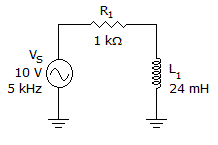# Electronics - RL Circuits - Discussion

### Discussion :: RL Circuits - Filling the Blanks (Q.No.2)

2.The phase angle in the circuit in the given circuit is approximately equal to ______.

 [A]. 53° [B]. 90° [C]. 37° [D]. 0°

Explanation:

No answer description available for this question.

 Vishnu said: (Aug 22, 2011) Phase angle = cos inverse((1k)^2/((1k^2)+(2*3.142*5k*0.024)^2)^0.5 = 37.015

 Manmeeth said: (Apr 9, 2012) Phase angle = tan(wL/R)

 Monica said: (Apr 25, 2012) XL = 2*(3.14)*f*L =2*3.14*5*10^3*24*10^-3 =240(3.14) Total phase angle= tan inverse(XL/ R) = tan inverse(240*(3.14)/10^3) =37.015

 Srijith Raju said: (Jun 9, 2012) tan (q) = XL/R q = Phase Angle (deg) XL = Inductance Reactance(ohm) R = Resistance (ohm)

 Shahid said: (Sep 26, 2012) Phase angle=tan^-1(wL/R) =tan^-1(2*3.14*24*10^-3/1*10^-3) =37

 Dharani said: (Feb 22, 2014) Phase angle = tan^-1(wL/R). = tan^-1(2*3.14*5*10^3*24*10^-3/1*10^3). = 37.

 Shivany said: (Mar 10, 2015) How to calculate inverse values? We do not have calculator in the exam halls right.

 S.Bala Kumar said: (May 19, 2015) I studied phase shift is tan inverse of (imaginary part/real part). Why that formula applied here?

 Shiv said: (Dec 4, 2016) This question asked in exam where calculator is allowed.

 Samurtza said: (Aug 31, 2017) Just keeping in mind the tangent graph and its relation in this circuit: (When Calculator is not allowed). tan(angle)=2*π*f*L/R; . For this question we can use the act==> angle is less than 45 if 0<(2*π*f*L/R)<1. Otherwise greater than that in positive values. Here we have (2*π*f*L/R) <1. Also, note that (2*π*f*L/R) will be positive always.